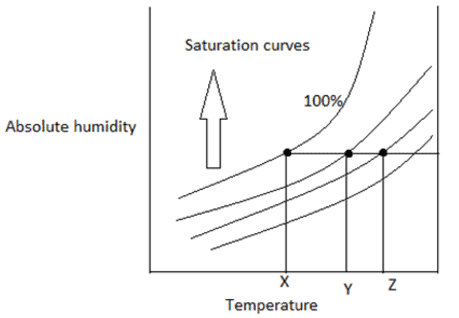Courses

# Test: Humidification Vapour Gas Mixture

## 10 Questions MCQ Test Mass Transfer | Test: Humidification Vapour Gas Mixture

Description
This mock test of Test: Humidification Vapour Gas Mixture for Chemical Engineering helps you for every Chemical Engineering entrance exam. This contains 10 Multiple Choice Questions for Chemical Engineering Test: Humidification Vapour Gas Mixture (mcq) to study with solutions a complete question bank. The solved questions answers in this Test: Humidification Vapour Gas Mixture quiz give you a good mix of easy questions and tough questions. Chemical Engineering students definitely take this Test: Humidification Vapour Gas Mixture exercise for a better result in the exam. You can find other Test: Humidification Vapour Gas Mixture extra questions, long questions & short questions for Chemical Engineering on EduRev as well by searching above.
QUESTION: 1

### Partial pressure equals vapour pressure if it is___________

Solution:

Explanation: At saturation, the vapour-gas mixture will be at equilibrium so the vapour pressure equals partial pressure.

QUESTION: 2

### Say True or False I) Vapour- at superheated temperature II) Gas – at condensation temperature

Solution:

Explanation: Since vapour has liquid portion we cannot say superheated. Superheated means free from other component like unique gas.

QUESTION: 3

### Guess the saturated molar absolute humidity of the gas(A.- Vapour(B. mixture at 100 degree Celsius. Vapour pressure of A is 300 mmHg and the total pressure is 760 mmHg.

Solution:

Explanation: Saturated molar humidity= Vapour pressure of A/ Vapour pressure of B
= 300/(760-300) =0.65.

QUESTION: 4

The system is unsaturated if partial pressure _________ equilibrium vapour pressure.

Solution:

Explanation: If saturated, the vapour pressure equals partial pressure. If unsaturated, the pressure will be less than vapour pressure.

QUESTION: 5

Consider a saturated gas(A.-vapour(B. mixture , if the absolute molal humidity is 0.265 and the total pressure= 760 mmHg. Find the vapour pressure of B.

Solution:

Explanation: First, we have to find the vapour pressure of A.
0.265= pA/760-pA
pA= 159.21
pB=760-159.21= 600.8.

QUESTION: 6

The vapour pressure of the nitrogen gas is 400mmHg and the partial pressure is 300mmHg. Find the relative saturation.

Solution:

Explanation: Relative saturation or relative humidity = (partial pressure/vapour pressure. x100
=75.

QUESTION: 7

Thermometer measurement temperature is

Solution:

Explanation: Dry bulb temperature is the actual measuring temperature of the thermometer.

QUESTION: 8

Find the dew pointSolution:

Explanation: The point at 100% saturation is known as dew point. Below the point, the vapour starts to condense.

QUESTION: 9

Temperature at which the partial pressure of the gas-vapour mixture equals vapour pressure _______

Solution:

Explanation: At saturation, the vapour pressure equals partial pressure. At dew point, saturation attains.

QUESTION: 10

Check whether we get approximately 80% saturation if the total pressure and the partial pressure of the gas mixture is 760 mmHg and 260 mmHg. Also the vapour pressure is 300 mmHg.

Solution:

Explanation: Percentage saturation is the ratio of absolute molal humidity to the saturated molal humidity. Absolute molal humidity= 260/(760-260. =0.52. Saturated molal humidity= 300/(760-300 =0.652.
%saturation= 0.52/0.652= 79.8(Approximately 80.